Международная студенческая научно-практическая конференция «Инновационное развитие государства: проблемы и перспективы глазам молодых ученых». Том 3

Baboshkin I.I., Kuzmenko V.І., Аtаnоvа М.Y.

Oles Honchar Dnipropetrovsk National University, Ukraine

RESEARCH OF MASSIVE STRUCTURES’ INFLUENCE ON HETEROGENEOUS BASES

Introduction

The necessity of making accurate estimations of buildings’ interaction with foundations arises in connection with intensification of building and regular building areas deficit. Nowadays building must be carried out on heterogeneous bases. That is why it is very important to carry out a research of non-uniform settlings which appears as the result of bases’ heterogeneity.

The main aim of this paper is to develop the models of non-uniform settlings of massive stamps to get deflected mode of bases using the contact problems theory for elastic solid.

1. Formulation of the problem

Plane deformation of heterogeneous band is being researched. The properties of the band are described by Lamé parameters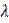and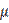in each point of the band. The band is being deformed under the influence of the massive stamp with a flat foundation. As a result of the band deformation the stamp receives vertical shift (settling)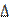and rotation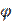, that are considered to be given (Fig. 1).

Let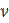be the unit vector of outer normal to the band surface. Let the surfaces of the band and stamp be coupled. For further let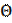be dilatational strain: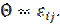Therefore the problem lies in finding components of displacement vector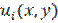, small deformation tensor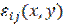and stress tensor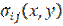.

Mentioned characteristics have to satisfy equations of equilibrium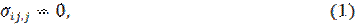Cauchy relations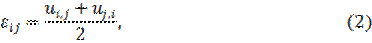determinative relations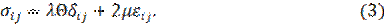Moreover, conditions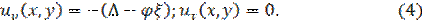must be satisfied on contact area of the stamp and the band.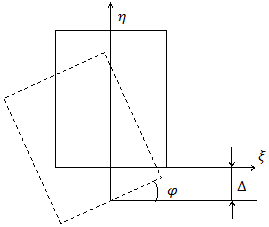Fig. 1. Rotation and shift of the stamp

1. Variational problem formulation

Specified problem formulation is unsuitable for construction of numerical methods solution. The most natural is its variational formulation. Variational method allows us to use developed methods of discretization. The typical method of variational problem formulation is considered in .

In order to make a variational problem formulation we are going to introduce the set of allowable functions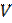. In this set we will include all allowable displacement vectors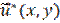, which components are continuous functions and they have continuous partial derivatives and satisfy condition (4) on contact area with the band.

For the specified problem we have received extremal variational problem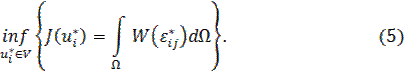It means that we must find a valid displacement among all of the motions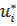from the allowable setfor which the minimal value of the functional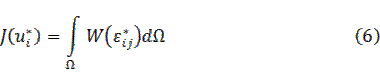corresponds to.

2. Discretization of the received problem

For discretization of the problem we use finite element method (FEM) that is the most widely-spread at the moment. The characteristic zones of the band for building finite-element mesh are shown on the Figure 2. As the finite elements we will take rectangular FE with bilinear approximation of allowable function. Motions are linearly distributed on the boundaries of the finite element so it provides continuity of the motions on its boundaries.

After the substitution of expressions for displacement into the functional (6) we will receive a multivariable function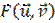. We have received a problem of unconstrained optimization of the multivariable function. We will get a linear system of equations by using the necessary condition of extremum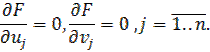Fig. 2. Characteristic zones of the band for building finite-element mesh

3. Method of solution of received linear system

The matrix of the linear system that we would receive using FEM is very discharged. Iterative methods are very effective for such systems. The method of successive over-relaxation (SOR) is one of the most efficient numerical methods. This method is a variant of the Gauss–Seidel method and it allows us to substantially increase the speed of convergence of the iterative process. The detailed description of the SOR method is given in .

It is proven that for systems with positive-definite symmetrical matrix relaxation parameter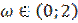leads to convergence of iterative process. When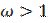the method is called method of upper relaxation. The speed of iterative process convergence can be changed by choosing relaxation parameter value. That is why we can say about the optimal value of the relaxation parameter. It is known that there is optimal value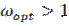of the relaxation parameter for systems that have been received within method of SOR. In practice values of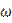near the optimal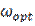are being searched within computing experiment.

As a stopping criterion of the iterative process can be taken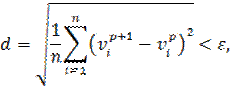where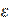is a given constant that is called error parameter.

The specified problem was solved within the software that was created. It was found out that for this class of problems the optimal value of relaxation parameter can be taken as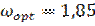. The practical convergence of approximated solutions was shown.